#0
0
01. 云栖社区>
2. 博客>
3. 正文

## 如何用Python和机器学习训练中文文本情感分类模型？# 疑惑# 数据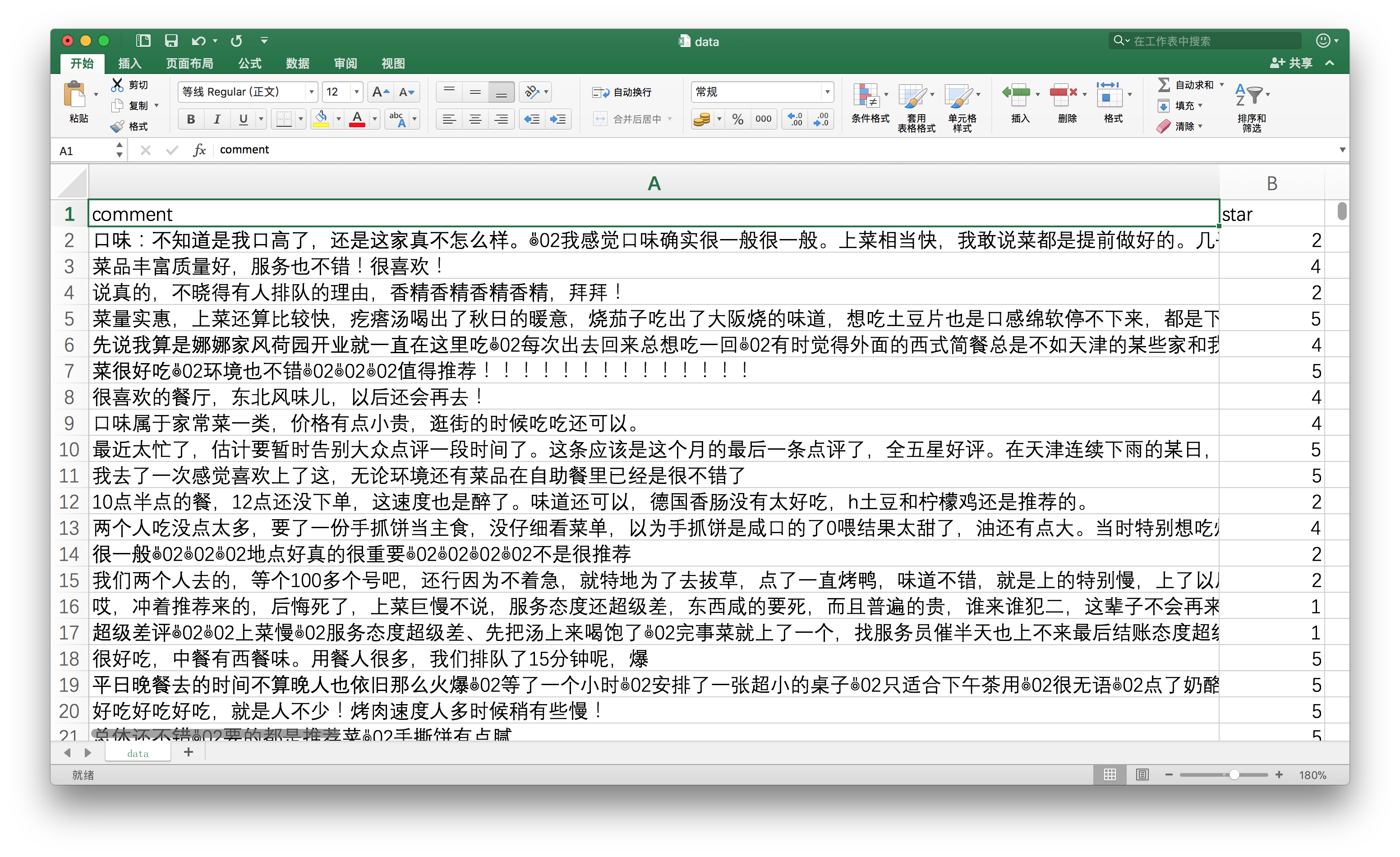# 模型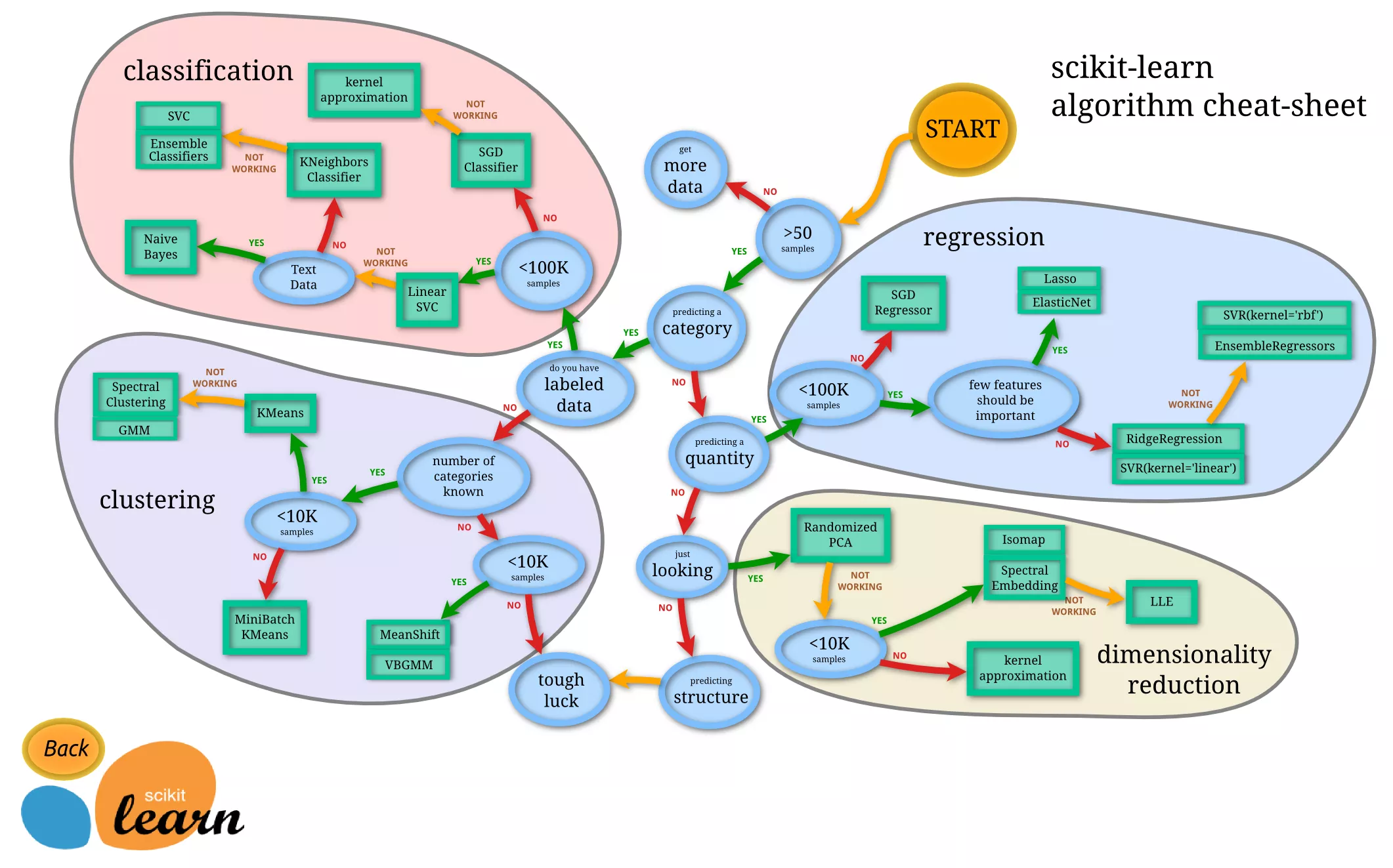Scikit-learn告诉我们：用朴素贝叶斯模型好了。

# 向量化

I love the game.

I hate the game.

• I
• love
• hate
• the
• game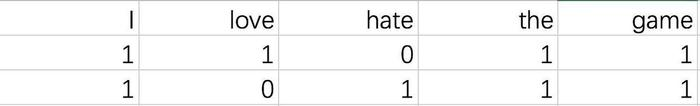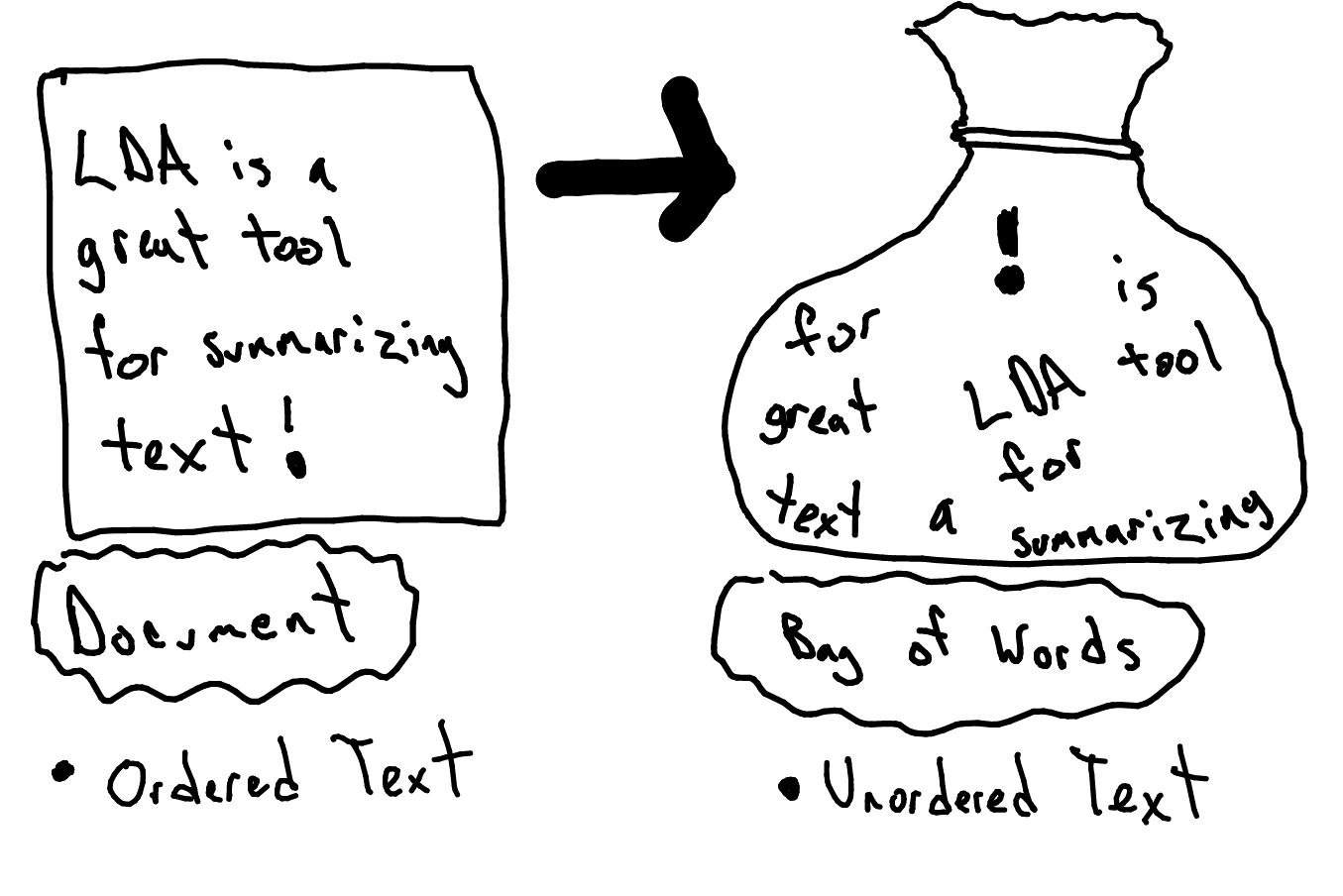# 中文

“我喜欢这个游戏”

“我 喜欢 这个 游戏”

I love the game.

I hate the game.

“我 喜欢 这个 游戏”

scikit-learn开发团队里，大概缺少足够多的中文使用者吧。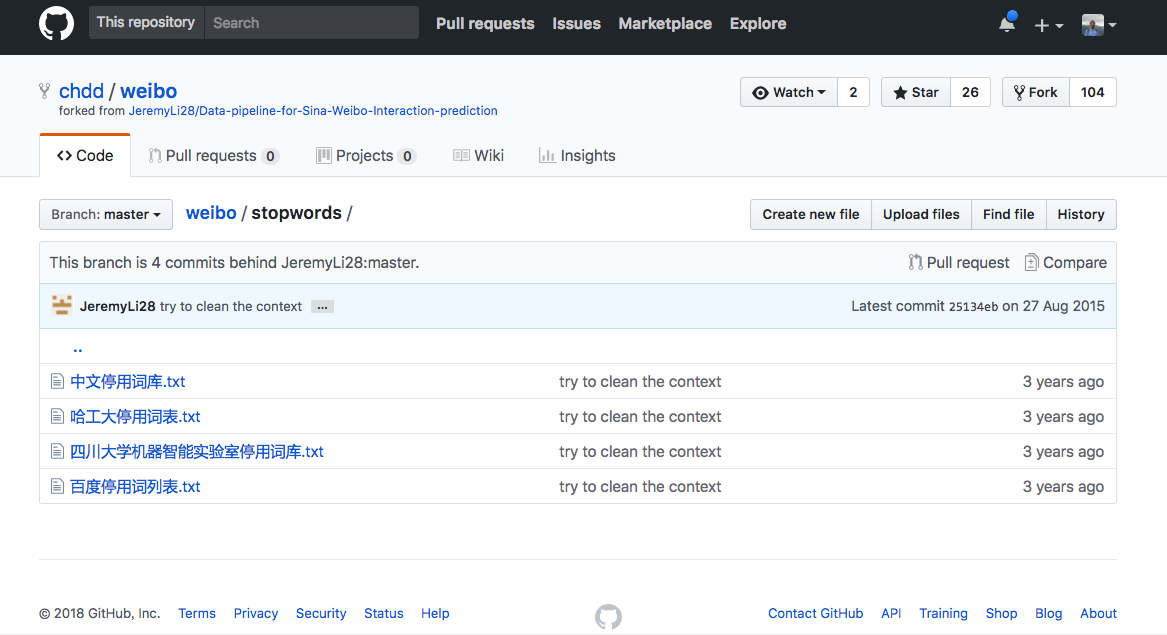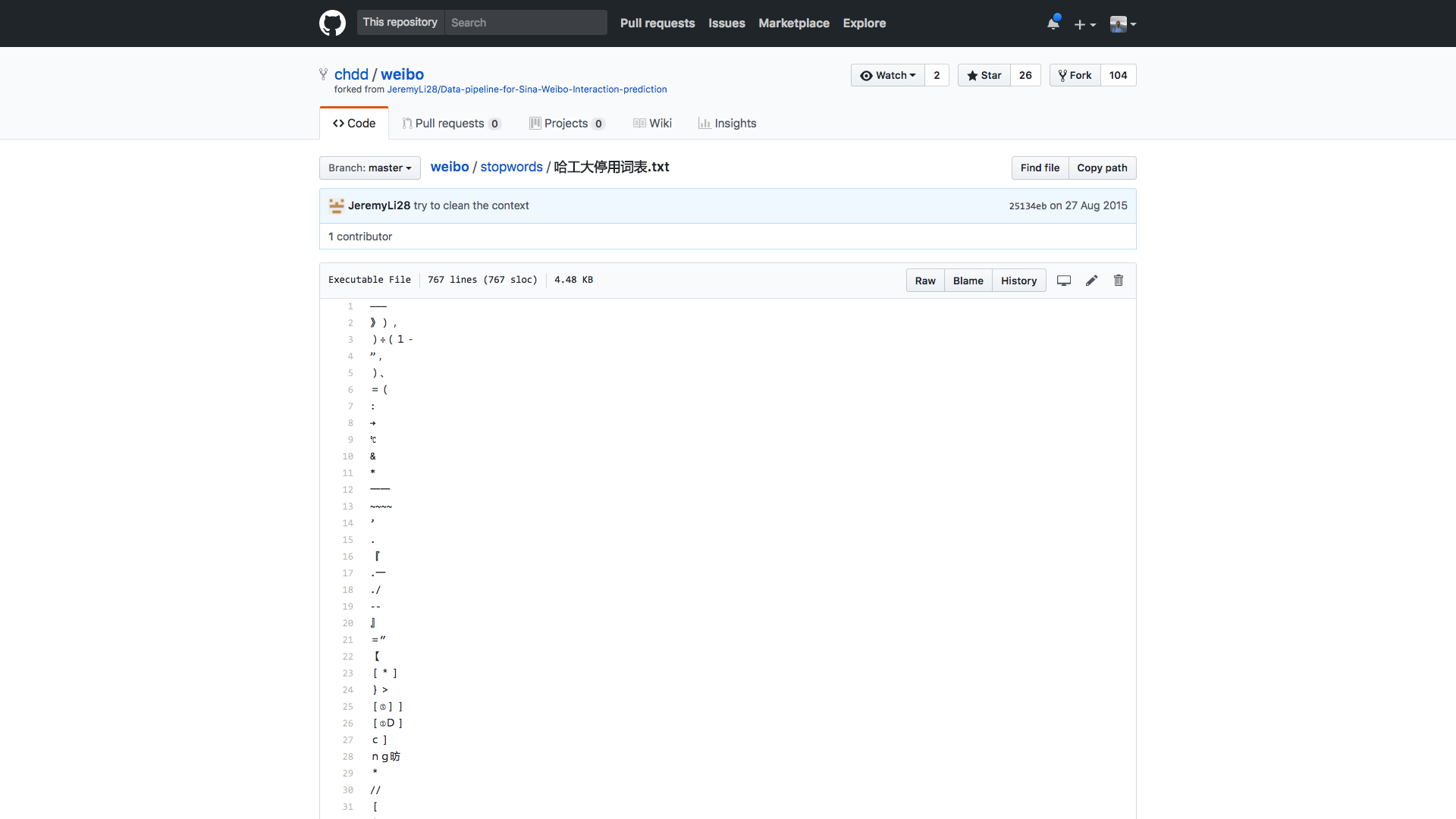# 环境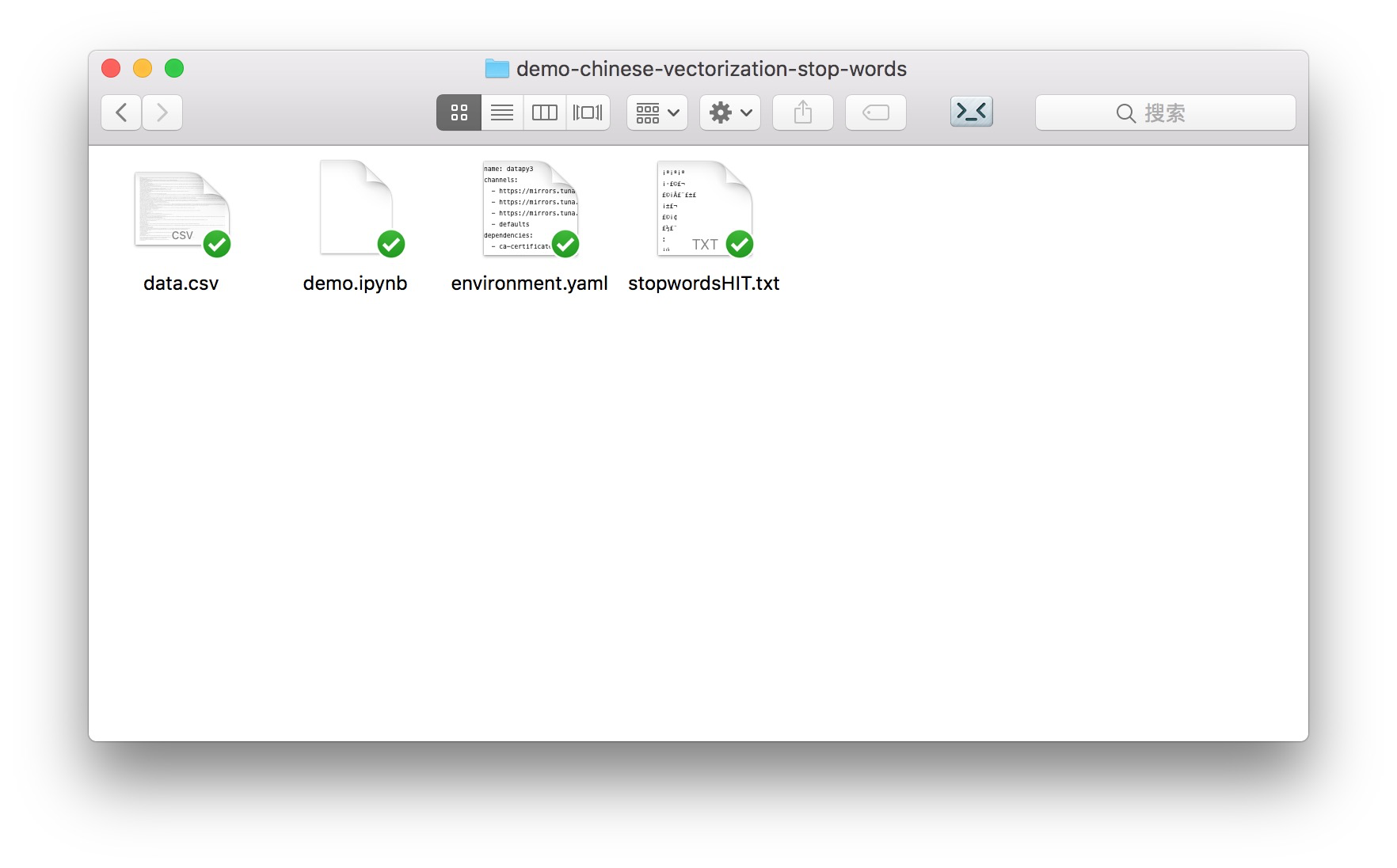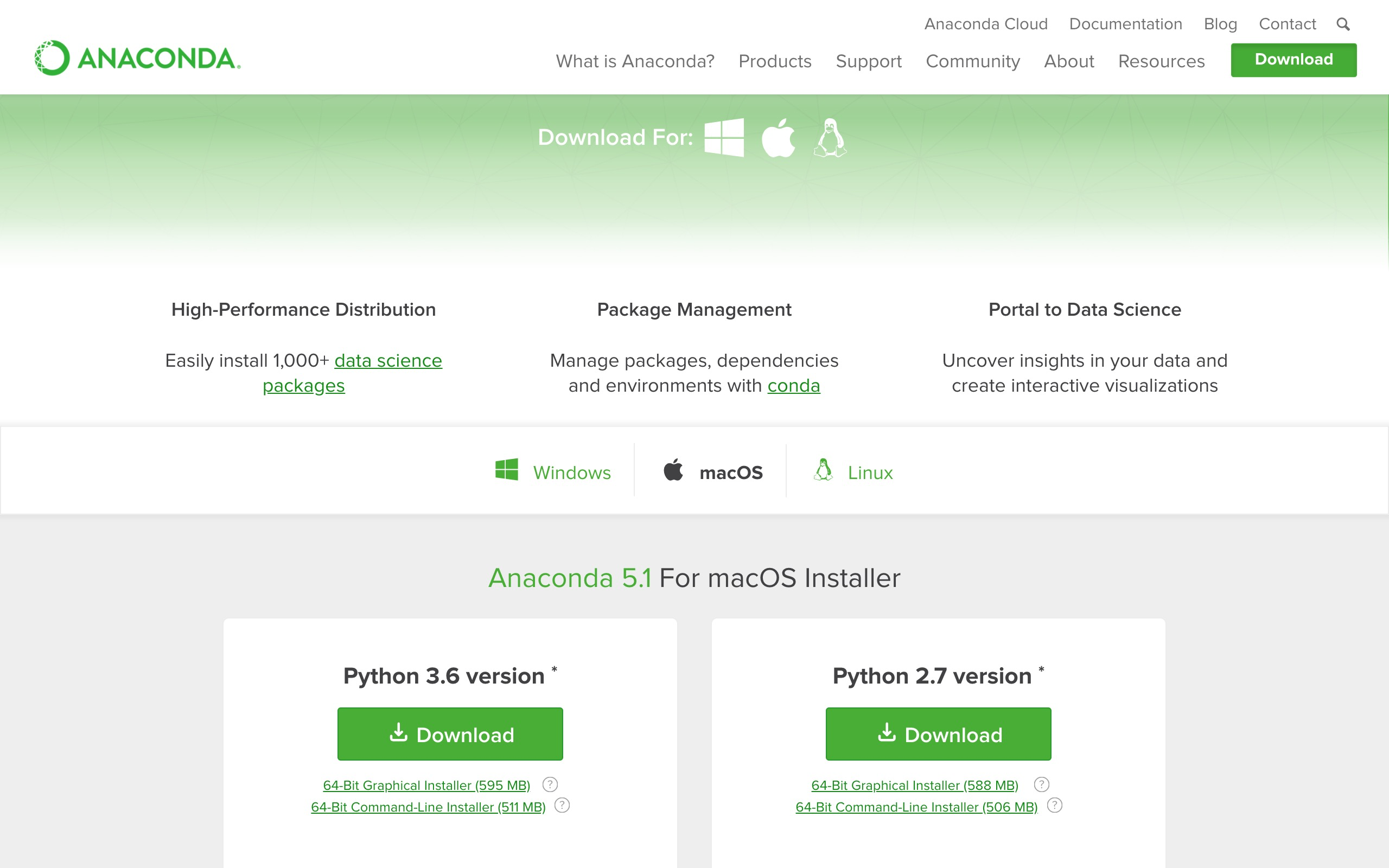conda env create -f environment.yaml

source activate datapy3

python -m ipykernel install --user --name=datapy3

jupyter notebook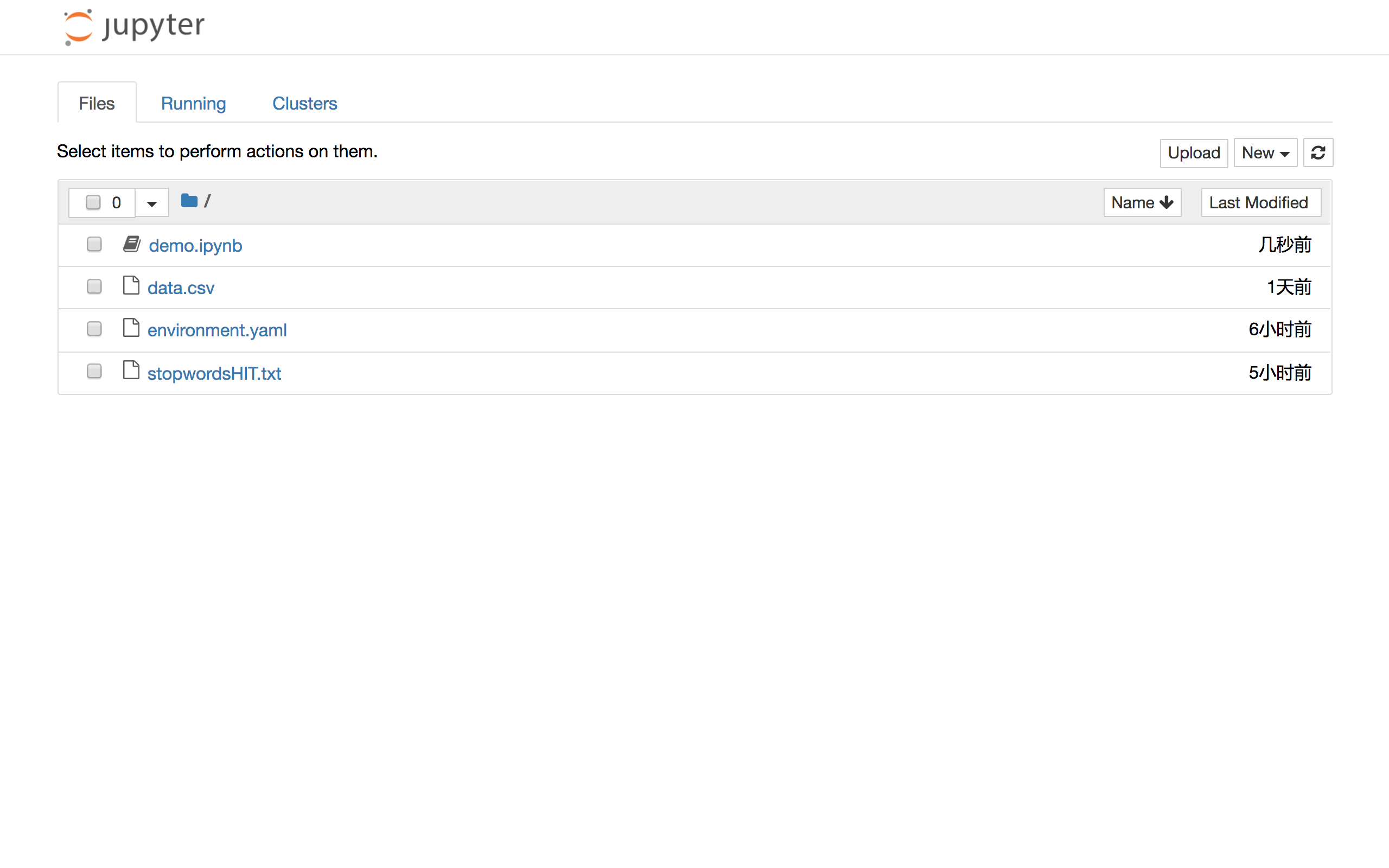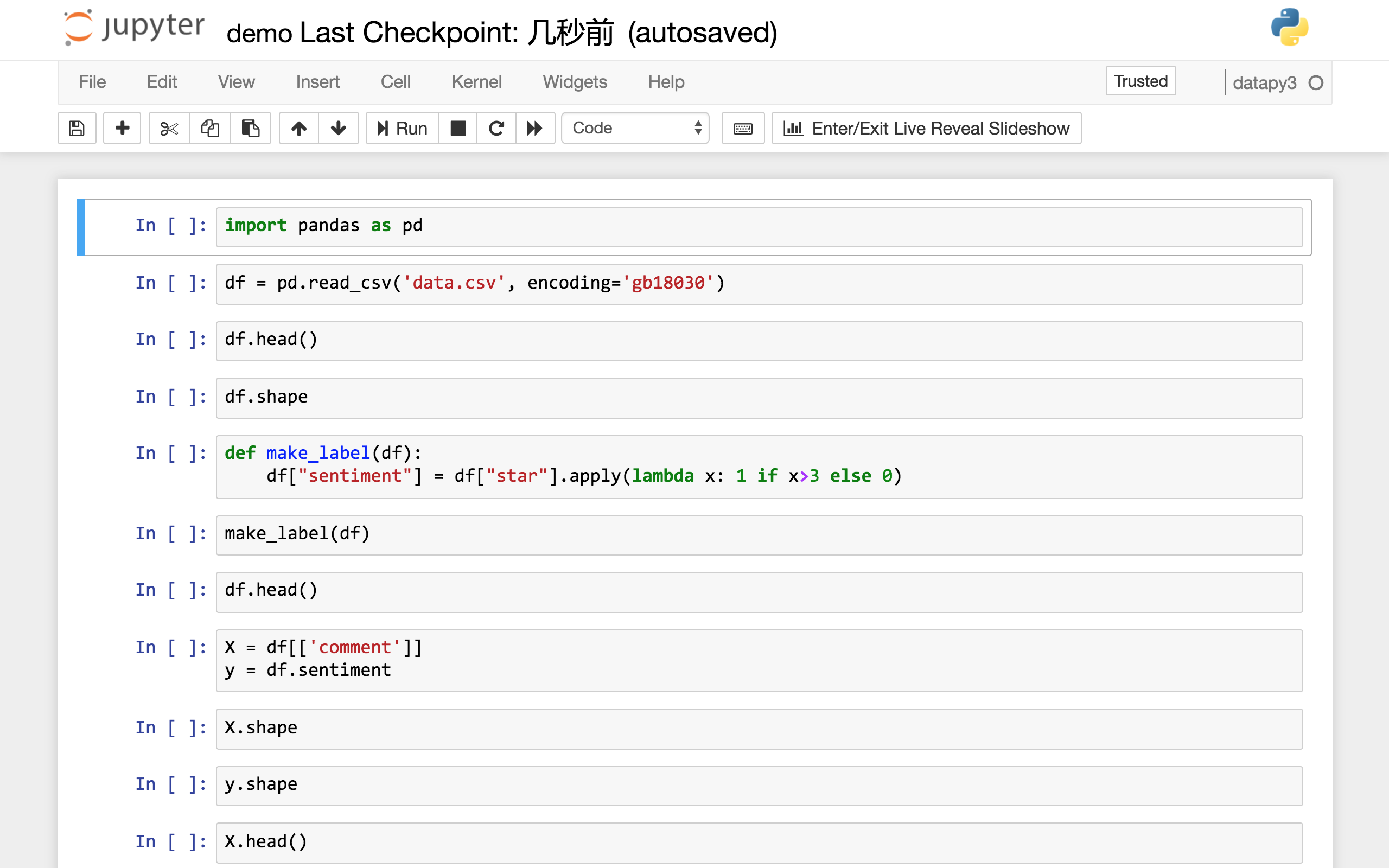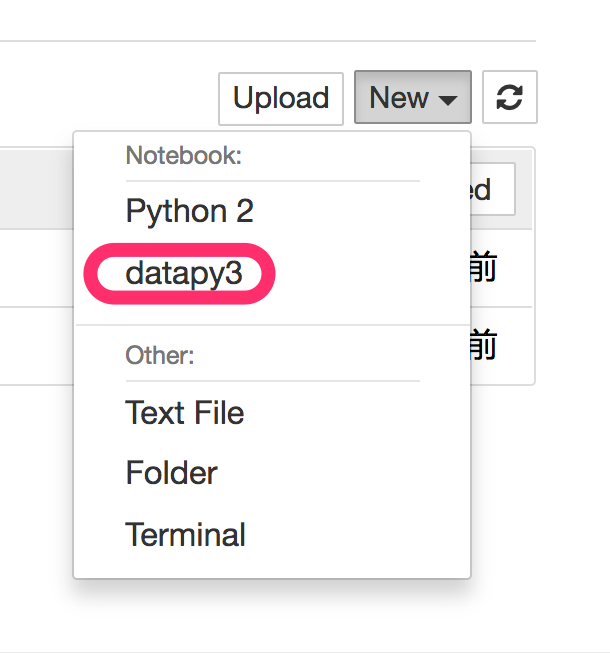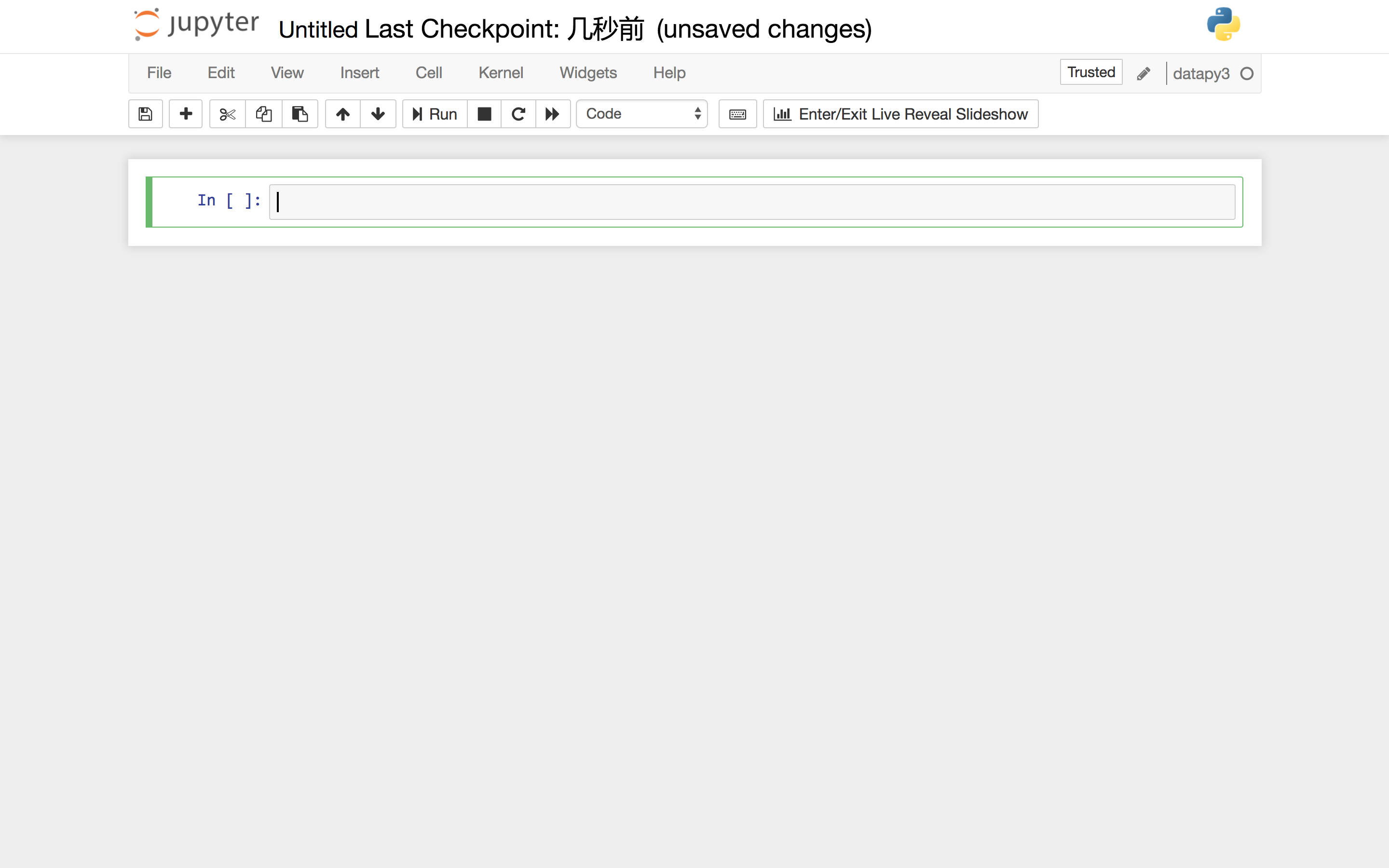# 代码

``````import pandas as pd
``````

``````df = pd.read_csv('data.csv', encoding='gb18030')
``````

``````df.head()
``````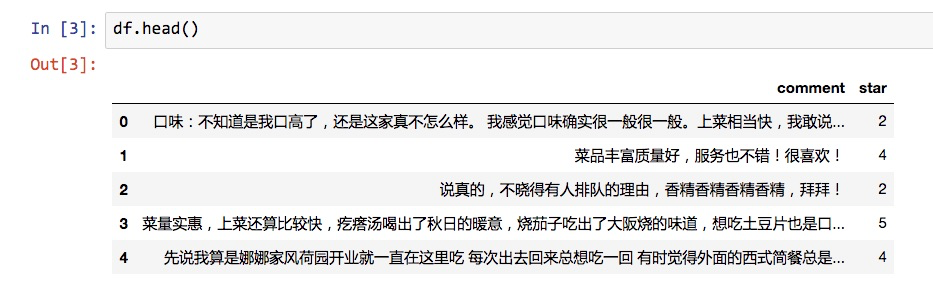``````df.shape
``````
``````(2000, 2)
``````

``````def make_label(df):
df["sentiment"] = df["star"].apply(lambda x: 1 if x>3 else 0)
``````

``````make_label(df)
``````

``````df.head()
``````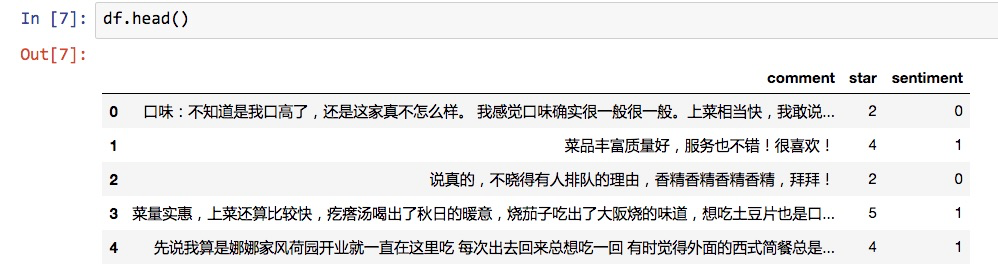``````X = df[['comment']]
y = df.sentiment
``````

X 是我们的全部特征。因为我们只用文本判断情感，所以X实际上只有1列。

``````X.shape
``````
``````(2000, 1)
``````

``````y.shape
``````
``````(2000,)
``````

``````X.head()
``````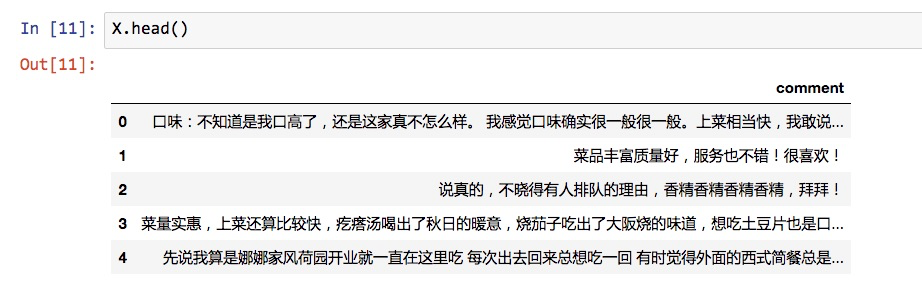``````import jieba
``````

``````def chinese_word_cut(mytext):
return " ".join(jieba.cut(mytext))
``````

``````X['cutted_comment'] = X.comment.apply(chinese_word_cut)
``````

``````X.cutted_comment[:5]
``````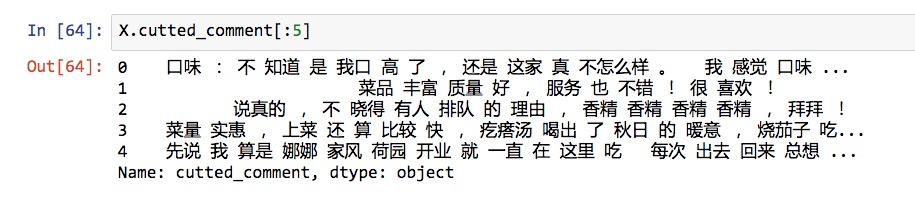``````from sklearn.model_selection import train_test_split
X_train, X_test, y_train, y_test = train_test_split(X, y, random_state=1)
``````

``````X_train.shape
``````
``````(1500, 2)
``````

``````y_train.shape
``````
``````(1500,)
``````
``````X_test.shape
``````
``````(500, 2)
``````
``````y_test.shape
``````
``````(500,)
``````

``````def get_custom_stopwords(stop_words_file):
with open(stop_words_file) as f:
stopwords_list = stopwords.split('\n')
custom_stopwords_list = [i for i in stopwords_list]
return custom_stopwords_list
``````

``````stop_words_file = "stopwordsHIT.txt"
stopwords = get_custom_stopwords(stop_words_file)
``````

``````stopwords[-10:]
``````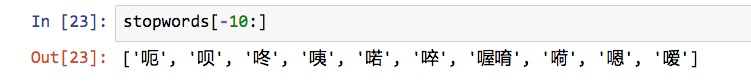``````from sklearn.feature_extraction.text import CountVectorizer
``````

``````vect = CountVectorizer()
``````

``````term_matrix = pd.DataFrame(vect.fit_transform(X_train.cutted_comment).toarray(), columns=vect.get_feature_names())
``````

``````term_matrix.head()
``````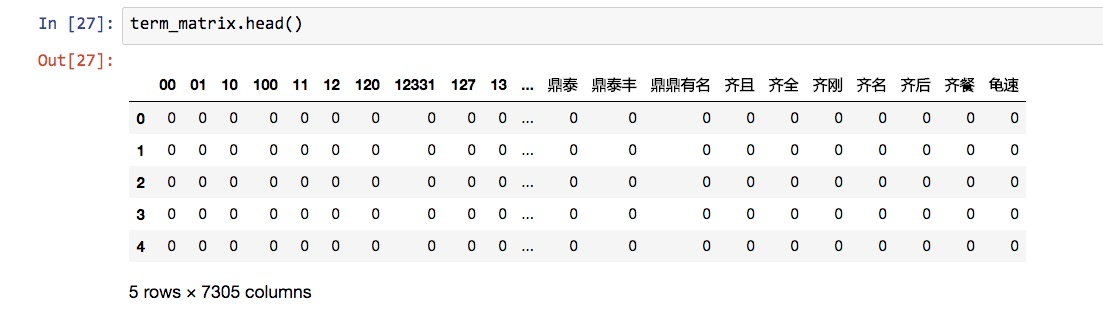`term_matrix`的形状如下：

``````term_matrix.shape
``````
``````(1500, 7305)
``````

``````vect = CountVectorizer(stop_words=frozenset(stopwords))
``````

``````term_matrix = pd.DataFrame(vect.fit_transform(X_train.cutted_comment).toarray(), columns=vect.get_feature_names())
``````
``````term_matrix.head()
``````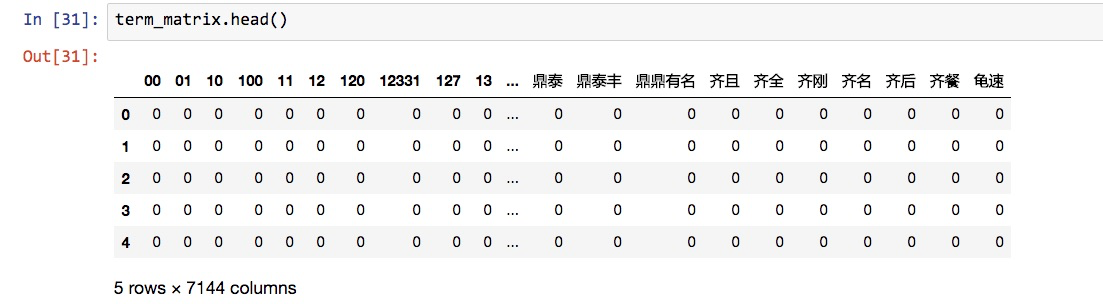``````max_df = 0.8 # 在超过这一比例的文档中出现的关键词（过于平凡），去除掉。
min_df = 3 # 在低于这一数量的文档中出现的关键词（过于独特），去除掉。
``````
``````vect = CountVectorizer(max_df = max_df,
min_df = min_df,
token_pattern=u'(?u)\\b[^\\d\\W]\\w+\\b',
stop_words=frozenset(stopwords))
``````

``````term_matrix = pd.DataFrame(vect.fit_transform(X_train.cutted_comment).toarray(), columns=vect.get_feature_names())
``````
``````term_matrix.head()
``````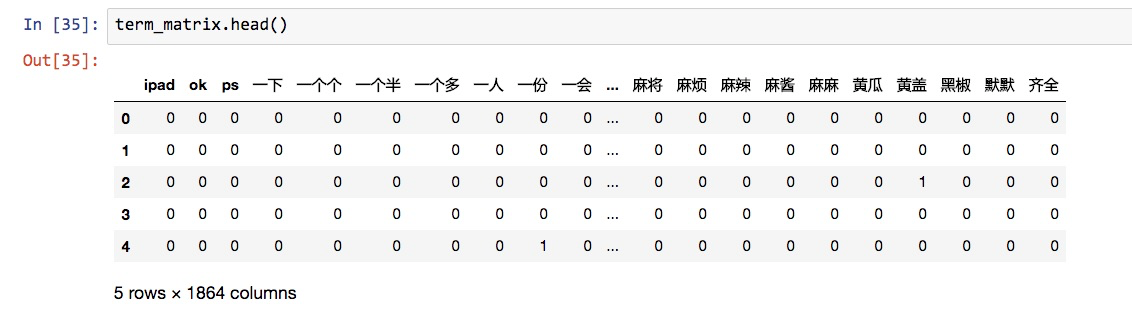``````from sklearn.naive_bayes import MultinomialNB
nb = MultinomialNB()
``````

1. 特征向量化；
2. 朴素贝叶斯分类。

``````from sklearn.pipeline import make_pipeline
pipe = make_pipeline(vect, nb)
``````

``````pipe.steps
``````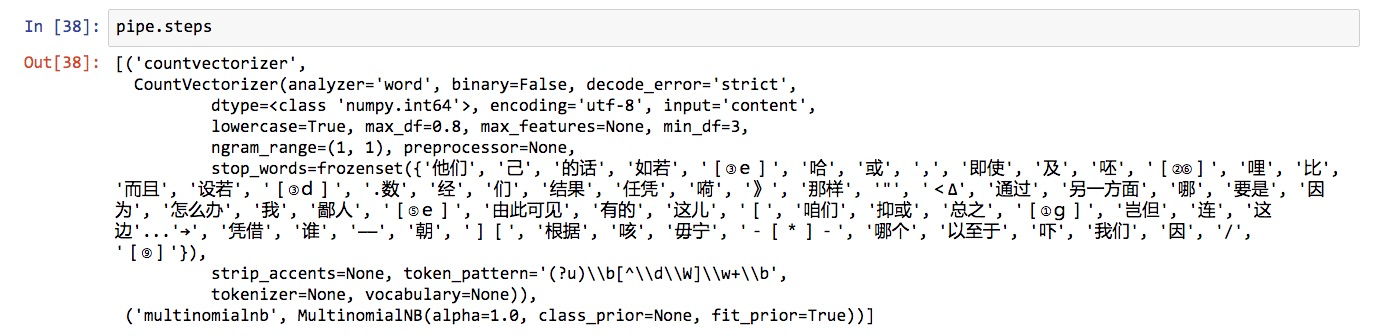``````from sklearn.cross_validation import cross_val_score
cross_val_score(pipe, X_train.cutted_comment, y_train, cv=5, scoring='accuracy').mean()
``````

``````0.820687244673089
``````

``````pipe.fit(X_train.cutted_comment, y_train)
``````

``````pipe.predict(X_test.cutted_comment)
``````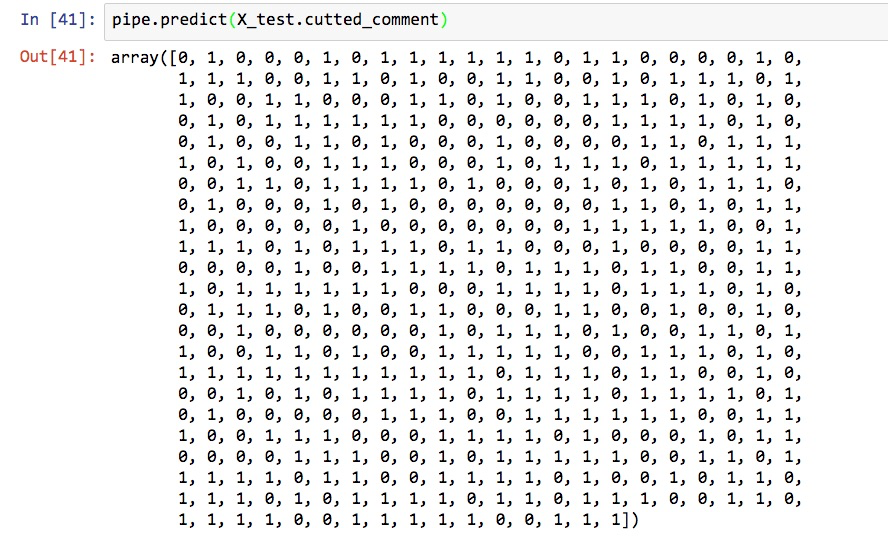``````y_pred = pipe.predict(X_test.cutted_comment)
``````

``````from sklearn import metrics
``````

``````metrics.accuracy_score(y_test, y_pred)
``````
``````0.86
``````

``````metrics.confusion_matrix(y_test, y_pred)
``````
``````array([[194,  43],
[ 27, 236]])
``````

• TP: 本来是正向，预测也是正向的；
• FP: 本来是负向，预测却是正向的；
• FN: 本来是正向，预测却是负向的；
• TN: 本来是负向，预测也是负向的。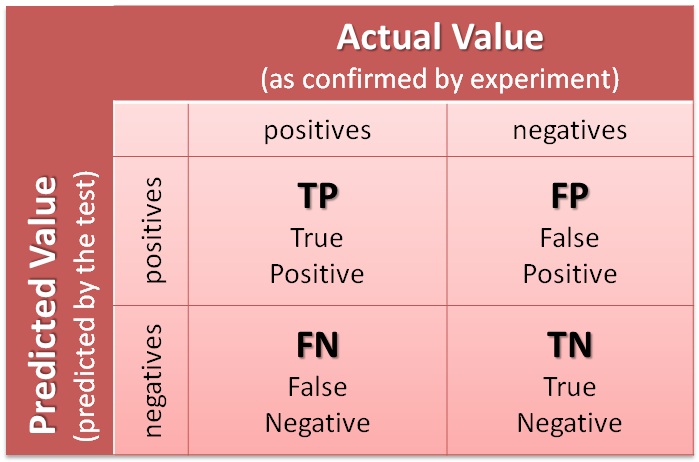``````from snownlp import SnowNLP
def get_sentiment(text):
return SnowNLP(text).sentiments
``````

``````y_pred_snownlp = X_test.comment.apply(get_sentiment)
``````

``````y_pred_snownlp_normalized = y_pred_snownlp.apply(lambda x: 1 if x>0.5 else 0)
``````

``````y_pred_snownlp_normalized[:5]
``````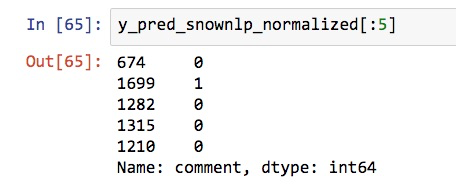``````metrics.accuracy_score(y_test, y_pred_snownlp_normalized)
``````
``````0.77
``````

``````metrics.confusion_matrix(y_test, y_pred_snownlp_normalized)
``````
``````array([[189,  48],
[ 67, 196]])
``````

# 小结

1. 如何用一袋子词（bag of words）模型将自然语言语句向量化，形成特征矩阵；
2. 如何利用停用词表、词频阈值和标记模式(token pattern)移除不想干的伪特征词汇，降低模型复杂度。
3. 如何选用合适的机器学习分类模型，对词语特征矩阵做出分类；
4. 如何用管道模式，归并和简化机器学习步骤流程；
5. 如何选择合适的性能测度工具，对模型的效能进行评估和对比。

+ 关注